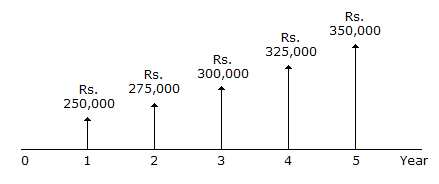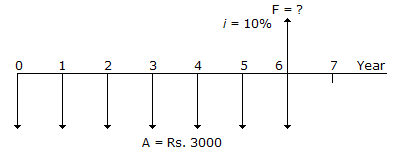# Civil Engineering - Engineering Economy

### Exercise :: Engineering Economy - Section 1

6.

Pick up the correct statement from the following:

 A. The change in the amount of money over a given time period is called 'time value' of money, a most important concept in engineering economy. B. The manifestation of the time value of money is termed as interest. C. Interest on borrowing = present amount owed - original loan D. The original investment (or loan) is referred to as principal. E. All of these.

Explanation:

No answer description available for this question. Let us discuss.

7.

Which one of the following is included in financial ratios of the firm ?

 A. Profitability ratio B. Liquidity ratio C. Turnover ratio D. Ratio of overall performances E. All of these

Explanation:

No answer description available for this question. Let us discuss.

8.

Pick up the correct statement from the following:

 A. The financial ratio summarises some aspect of the firm's financial condition at the time of preparing a balance sheet. B. Both the numerator and denominator of financial ratios come directly from the balance sheet. C. Income statement ratios compare one 'flow' item from the income statement to another flow item from the income statement. D. Income statement ratios compare a flow item from the income statement to another flow item form the income statement E. All of these

Explanation:

No answer description available for this question. Let us discuss.

9.

Refer to the cash flow diagram of uniform gradient in a cash flow (in the given figure), the gradient is :A. Rs 10000 per year B. Rs 15000 per year C. Rs 20000 per year D. Rs 25000 per year

Explanation:

No answer description available for this question. Let us discuss.

10.

In the cash-flow diagram shown in the given figureA. Equal deposits of Rs 3000 per year (A) are made, starting now. B. The rate of interest is 10% per yearaccount C. The amount accumulated after the seventh deposit is to be computed D. All of these